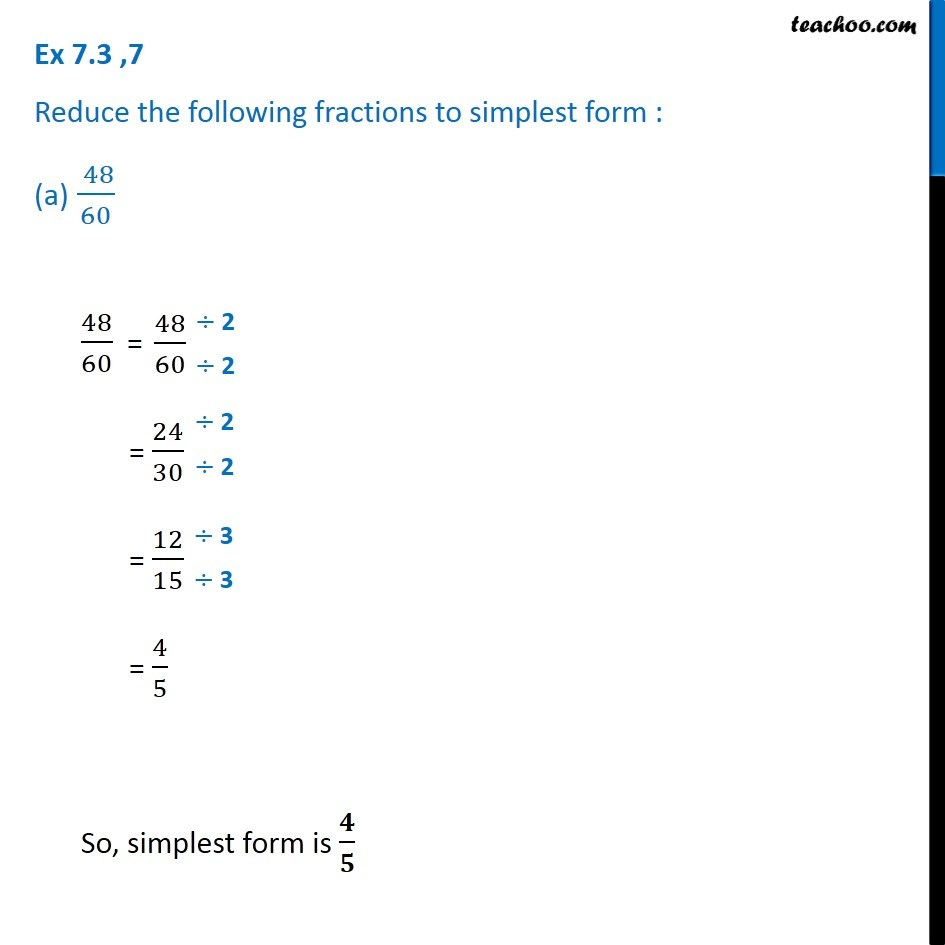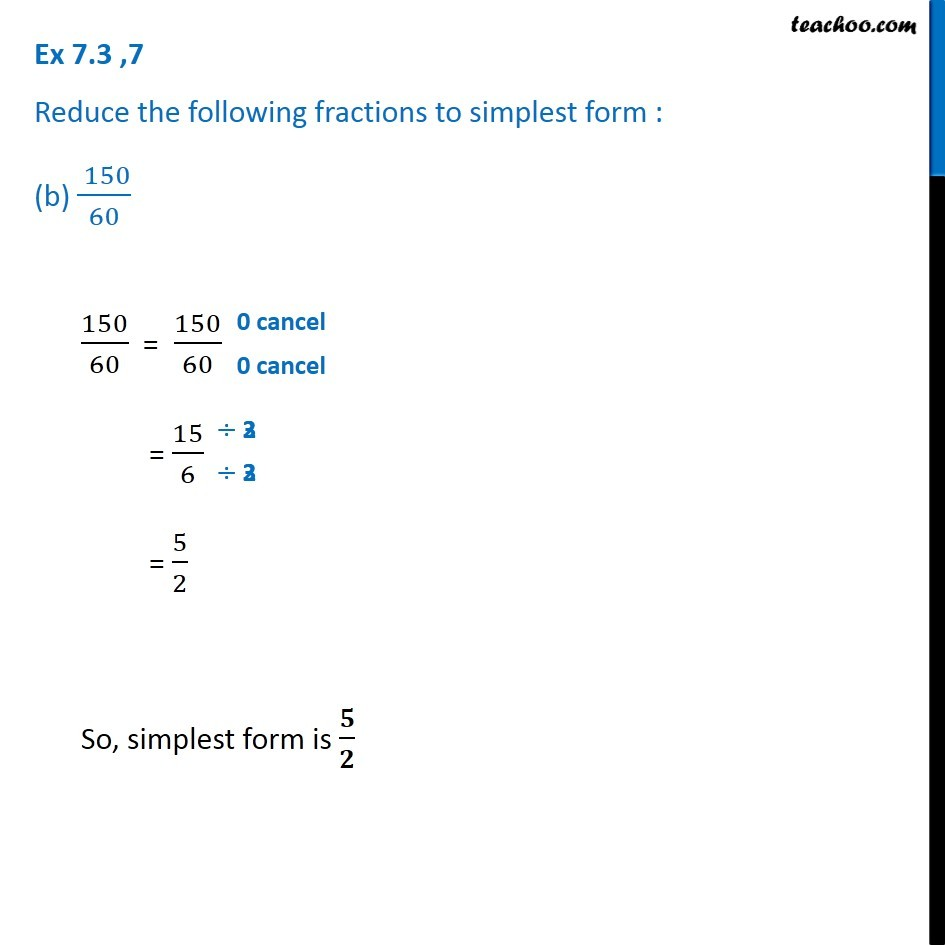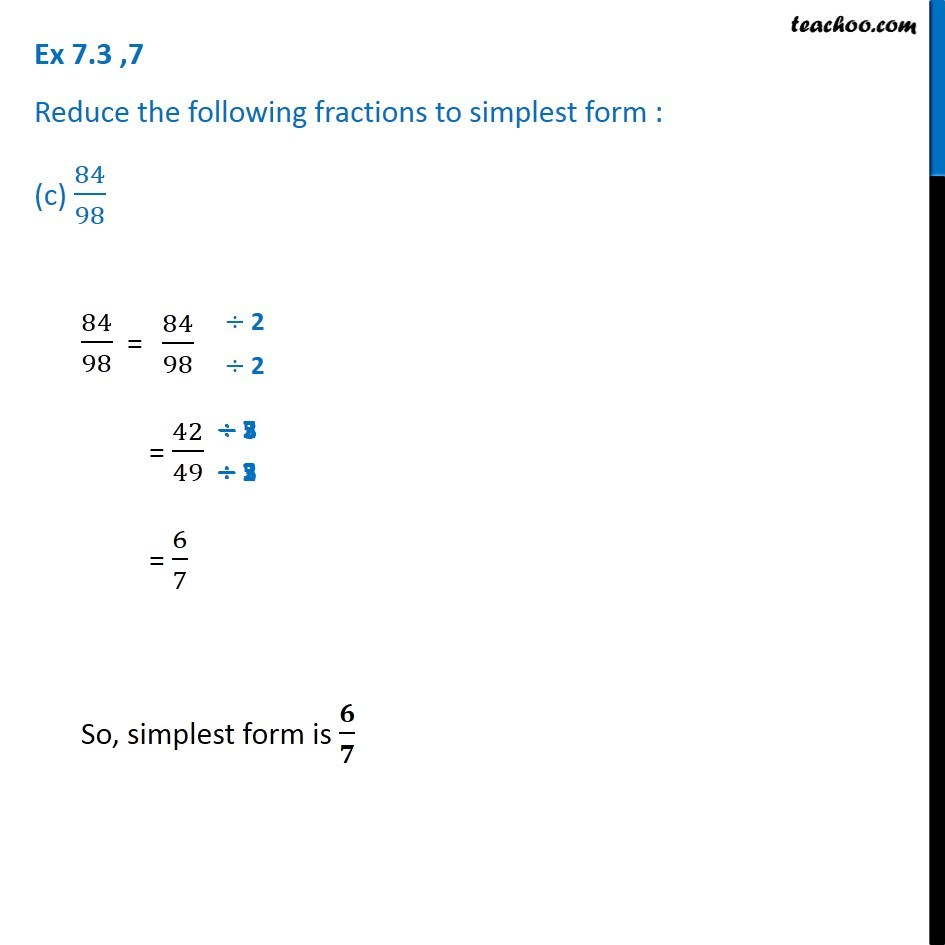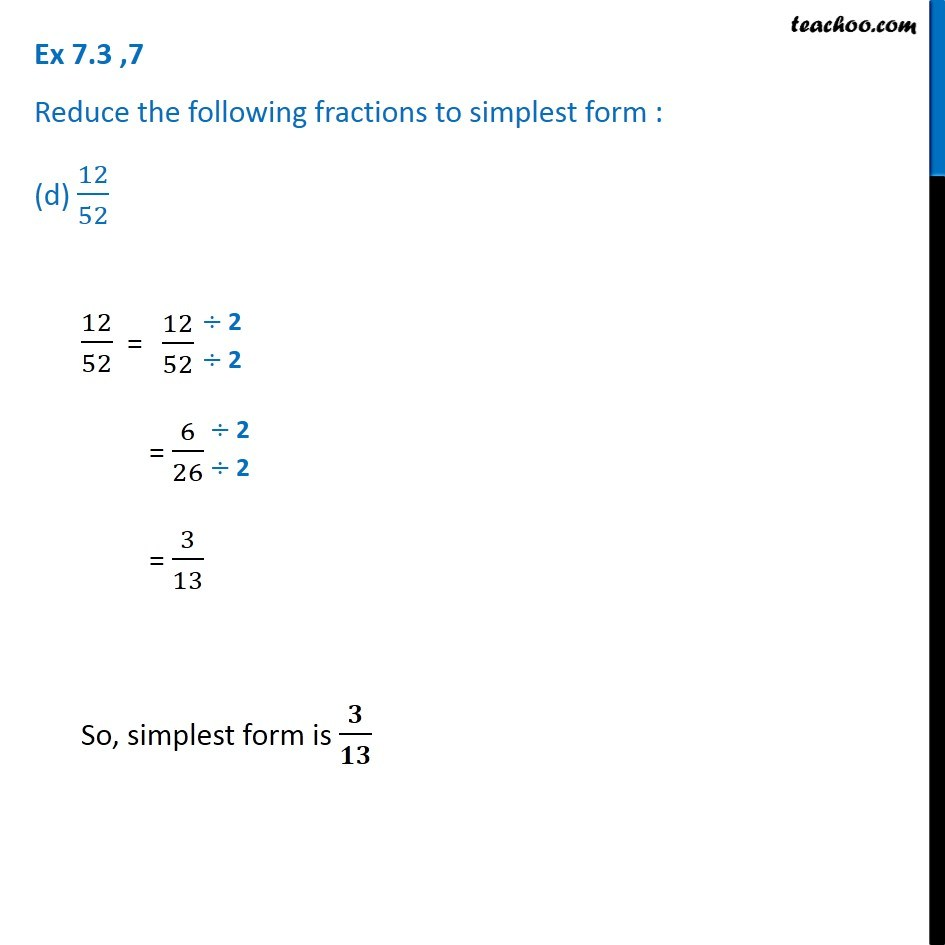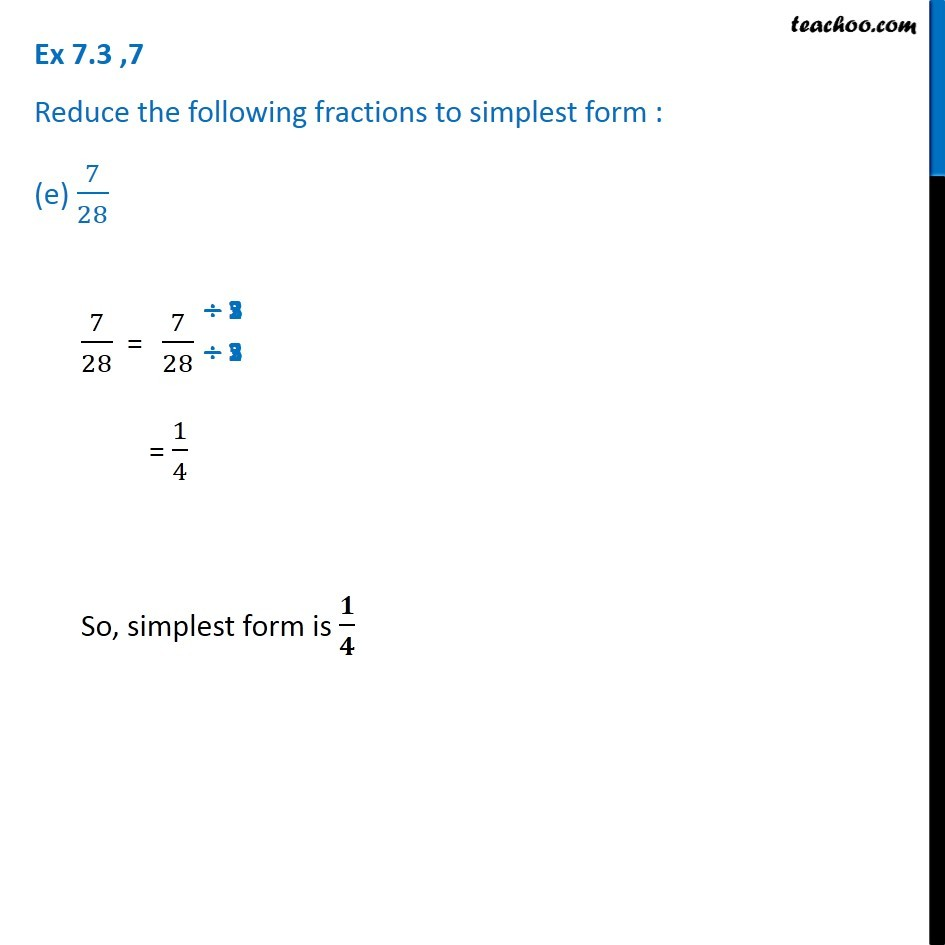Subscribe to our Youtube Channel - https://you.tube/teachoo

1. Chapter 7 Class 6 Fractions
2. Serial order wise
3. Ex 7.3

Transcript

Ex 7.3 ,7 Reduce the following fractions to simplest form : (a) ( 48)/6048/60 = = 24/30 = 12/15 = 4/5 So, simplest form is 𝟒/𝟓 Ex 7.3 ,7 Reduce the following fractions to simplest form : (b) ( 150)/60150/60 = = 15/6 = 5/2 So, simplest form is 𝟓/𝟐 Ex 7.3 ,7 Reduce the following fractions to simplest form : (c) 84/98 84/98 = = 42/49 = 6/7 So, simplest form is 𝟔/𝟕 Ex 7.3 ,7 Reduce the following fractions to simplest form : (d) 12/52 12/52 = = 6/26 = 3/13 So, simplest form is 𝟑/𝟏𝟑 Ex 7.3 ,7 Reduce the following fractions to simplest form : (e) 7/28 7/28 = = 1/4 So, simplest form is 𝟏/𝟒

Ex 7.3

Chapter 7 Class 6 Fractions
Serial order wise